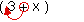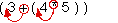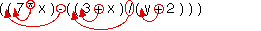Design Recipes

Recipe for converting infix algebraic expressions to Scheme notation

1. Expand abbreviations. For example,
3(x-2) actually means 3 * (x-2),
7x2 actually means 7 * x * x, etc.

2. Fully parenthesize the expression, even the outermost operator, so that (a) it is completely unambiguous which operation needs to happen next, and (b) every operator is surrounded by its own private pair of parentheses. For example,
3 + x becomes (3 + x),
3 + 4 * 5 becomes (3 + (4 * 5)),
7 * x - (3+x)/(y+2) becomes ((7 * x) - ((3 + x) / (y + 2))), etc.
Note that by the time you're done with this step, there should be the same number of left parentheses, right parentheses, and operators: in the last example, there are five operators (*, -, +, /, +) so there must be five left parentheses and five right parentheses.

3. Move each operator left to just after its left-parenthesis. For example,becomes (+ 3 x)becomes (+ 3 (* 4 5))becomes (- (* 7 x) (/ (+ 3 x) (+ y 2)))
etc. Be careful not to move anything except operators!